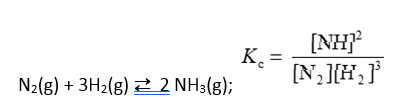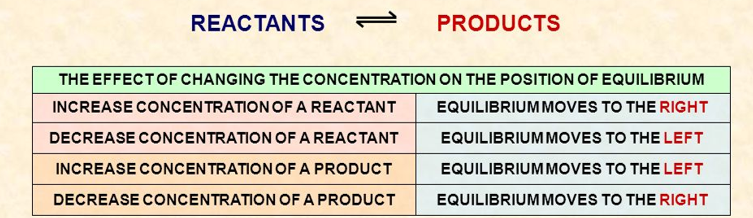# Factors affecting the equilibrium

Equilibrium: Factors affecting the equilibrium

The equilibrium of a system shift to a new position in order to minimize any changes due to the alteration of certain factors that influence the equilibrium. To determine which factors, affect the rate, it is important to determine what happens during a chemical reaction. In a chemical reaction, the particles of each of the reactants are colliding with one another and transferring energy. The speed of the reaction depends on the number of collisions and how effective those collisions are.Nature of the Reactants

Intermolecular bonds such as Metallic, Hydrogen, Ionic, Polar Covalent and Non-polar Covalent keep the constituents of the reaction intact. This means that the stronger the bond within reactants, the slower the reaction.

The concentration of the Reactants

As the concentration of the reactants increases, the frequency of collisions increases as does the rate. In a gas system, the greater the concentration, the greater the pressure; therefore, the increase in pressure leads to an increase in reaction rate

Consider the reaction:If the concentration of nitrogen or hydrogen is increased, the rate of the forward reaction is more than backward. A net forward reaction will occur to reach a new equilibrium position. If [NH3] is increased, a net reverse reaction will occur to come to a new equilibrium position.

Pressure

If the volume of a gas mixture is compressed, the overall gas pressure will increase. In which direction the equilibrium will shift in either direction depends on the reaction stoichiometry. However, there will be no effect to equilibrium if the total gas pressure is increased by adding an inert gas that is not part of the equilibrium system.

Consider the reaction:

2SO2(g) + O2(g) 2SO3(g)

The total moles of the gas decreases as reaction proceeds in the forward direction. If the pressure is increased by decreasing the volume (compression), a forward reaction occurs to reduce the stress. Reactions that result in fewer moles of gas favour high-pressure conditions.

Consider the following reactions that are not affected by pressure:

CO(g) + H2O(g) CO2(g) + H2(g);

H2(g) + Cl2(g) 2HCl(g);

Reactions have the same number of gas molecules in reactants and products. Reducing or increasing the volume will cause an equal effect on both sides – no net reaction will occur. This means that the equilibrium is not affected by the change in pressure.

Temperature

As the average kinetic energy increases, that would be the temperature, the number of collisions increases and therefore the rate of reaction increases. Temperature is the only factor that changes the equilibrium constant with its alterations.

Consider the following exothermic reaction:

N2(g) + 3H2(g) 2NH3(g); ∆Ho = -92 kJ,

The forward reaction produces heat => heat is a product. When heat is added in the system, the reverse reaction will take place to absorb the heat. Likewise, if heat is removed to reduce temperature, a net forward reaction will occur to produce heat. Accordingly, Exothermic reactions favour low-temperature conditions.

Surface Area

The greater the surface area, the greater the probability of surface collisions; therefore, the reaction rate increases

Catalysts

The presence of a catalyst lowers the activation energy required to initiate a reaction by providing an alternative pathway. This alternative pathway allows for a faster reaction rate.Figure 1: Summary of factors affecting the position of equilibrium.